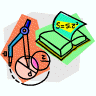## Mathematics, Department of## Faculty Publications, Department of Mathematics

2007

#### Citation

Published in Advances in Mathematics of Communications 1:1 (2007), pp 111-130. doi: 10.3934/amc.2007.1.111

Copyright 2007 American Institute of Mathematical Sciences

#### Abstract

Binary self-dual codes and additive self-dual codes over F4 have in common interesting properties, for example, Type I, Type II, shadows, etc. Recently Bachoc and Gaborit introduced the notion of s-extremality for binary self-dual codes, generalizing Elkies' study on the highest possible minimum weight of the shadows of binary self-dual codes. In this paper, we introduce a concept of s-extremality for additive self-dual codes over F4, give a bound on the length of these codes with even distance d, classify them up to minimum distance d = 4, give possible lengths and (shadow) weight enumerators for which there exist s-extremal codes with 5 ≤ d ≤ 11 and give five s-extremal codes with d = 7. We construct four s-extremal codes of length n = 13 and minimum distance d = 5. We relate an s-extremal code of length 3d to another s-extremal code of that length, and produce extremal Type II codes from s-extremal codes.

COinS